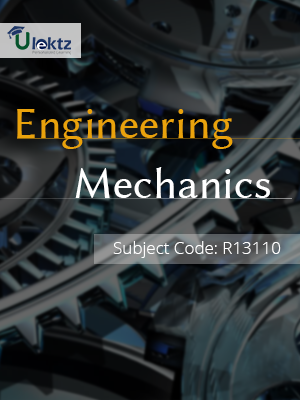uLektz apps

•My WalletMy Order
•My Profile
•My Connections
•My Books
•My Videos
•My Tests
•My Calender
•My Messages
•My Shopping Cart
•My Orders
•Account Settings
•Help

uLektz apps

Book DetailsEngineering Mechanics

Course Code:R13110

Author:uLektz

University:

Regulation:2016

Categories:Civil

Format :ePUB3 (DRM Protected)

Type :eBook

FREE

Description :Engineering Mechanics of R13110 covers the latest syllabus prescribed by JNTU Kakinada for regulation 2016. Author: uLektz, Published by uLektz Learning Solutions Private Limited.

Note : No printed book. Only ebook. Access eBook using uLektz apps for Android, iOS and Windows Desktop PC.

Topics
UNIT I SYSTEMS OF FORCES

1.1 Introduction to Engg.Mechanics – Basic Concepts.

1.2 Systems of Forces: Coplanar Concurrent Forces -Components in Space -Resultant

1.3 Moment of Force and its Application -Couples and Resultant of Force Systems

1.4 Introduction, limiting friction and impending motion, coulomb’s laws of dry friction, coefficient of friction, cone of friction.

UNIT II EQUILIBRIUM OF SYSTEMS OF FORCES

2.1 Equilibrium of Systems of Forces :Free Body Diagrams, Equations of Equilibrium of Coplanar Systems, Spatial Systems for concurrent forces.

2.2 Lami’s Theorm, Graphical method for the equilibrium of coplanar forces, Converse of the law of Triangle of forces, converse of the law of polygon of forces condition of equilibrium.

UNIT III Centroid And Centre of Gravity

3.1 Centroid :Centroids of simple figures (from basic principles ) -Centroids of Composite Figures

3.2 Centre of Gravity :Centre of gravity of simple body (from basis principles), centre of gravity of composite bodies, pappus theorem.

UNIT IV AREA MOMENTS OF INERTIA AND MASS MOMENT OF INERTIA

4.1 Area moments of Inertia: Definition – Polar Moment of Inertia-Transfer Theorem

4.2 Moments of Inertia of Composite Figures-Products of Inertia

4.3 Transfer Formula for Product of Inertia

4.4 Mass Moment of Inertia: Moment of Inertia of Masses-Transfer Formula for Mass Moments of Inertia, mass moment of inertia of composite bodies

UNIT V KINEMATICS AND KINETICS

5.1 Kinematics: Rectilinear and Curvilinear motions -Velocity and Acceleration-Motion of Rigid Body -Types and their Analysis in Planar Motion

5.2 Kinetics: Analysis as a Particle and Analysis as a Rigid Body in Translation -Central Force Motion -Equations of Plane Motion.

5.3 Fixed Axis Rotation -Rolling Bodies

UNIT VI WORK – ENERGY METHOD

6.1 Work – Energy Method: Equations for Translation-Work - Energy Applications to Particle Motion

6.2 Connected System- Fixed Axis Rotation and Plane Motion

6.3 Impulse momentum method

Related Books